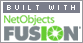Coming to Statistica
in 2022/2023

Group Classes

Interactive Webinars

Blog

Guestbook

.

E-mail        anne@statistica.com.au

# Place         Willetton, Western Australia# Mathematics & Statistic Tutor Perth - SPSS HelpRemember that mathematics questions are like sandwiches: First Slice of bread                                    Definitions i.e. Let x be ... The Filling                                                    The calculations Second Slice of bread                               Concluding sentence When you use a letter then you must define that letter at the beginning of the question. The answer should be able to be read without looking back at the question. So make sure that your sandwich is a Healthy Gourmet one and not a Stale Basic one!Example One Ross has \$61 and wants to divide the sum between Thomasine, Louis and Joshua. Thomasine has twice as much as Louis and Joshua has \$5 less than Thomasine and Louis together. How much do all three have each?Answer One Start off by defining the variables, now you can do this part in several ways so do the one that suits you. Let the amount Thomasine has be \$ TLet the amount Louis has be \$ LLet the amount Joshua has be \$ J From the question, we know that Thomasine has twice as much as Louis, T = 2L     So we can write 2L instead of T From the question, we know that Joshua has \$5 less than Thomasine and Louis together so, J = (T + L ) -  5  if we replace T by 2L we get, J = 2L + L - 5  = 3L -  5 So we now have all the amounts with just one letter which is what we want so that we can solve it.We didn't have to use three letters as we did above,  we could have used one letter from the beginning Let the amount Louis has be \$ L, so if Thomasine has twice as much as Louis then she has \$ 2L. If Joshua has \$5 less than Thomasine and Louis together then he has \$ (2L + L-  5 ) or  \$ 3L -  5 Either of these ways are fine just make sure that the person reading the answer knows what the letter or letters stand for.Back to the question, All three amounts add up to \$61 as this is the amount Ross has to share. 2L       +     L     +     (3L -  5)   =   61 Thomasine +  Louis +  Joshua    =  Total from RossCollecting all the letters and numbers on different sides 2L       +     L     +     3L  -   5   =   616L  -  5   =   61 We add all the letters together then add 5 to both sides -5 + 5 = 0 on the left-hand side  61 +5 on the right-hand side.                         6L   =   61 + 5 =  66Now we want L not 6L 6L   = 66 Dividing both sides by 6, we find that L = 11Now this is not the answer as you were asked for  “ How much do all three have each? ” 2L             +     L           +     (3L -  5) =   61 Thomasine    +  Louis     +  Joshua      = Total from Ross        \$22                  \$11         \$(33 -  5)                                                    \$28 That is the end of our calculations but not the end of the question! We must end with a sentence. Ross shares out \$61 with Thomasine receiving \$22, Louis receiving \$11 and Joshua receiving \$28. Back to School